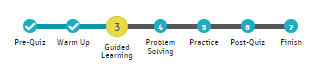# Lesson components in Imagine Math 3+

This article explains the parts of the lessons in Imagine Math 3+. The visual at the top of the student's screen indicates which part of the lesson they are on (in yellow) and which parts they've already completed (check marks).PRE-QUIZ: This activity is designed to assess student understanding of the content. Imagine Math does not provide feedback, Math Help, or access to Live Teachers in this activity. Students who earn at least 80% may be allowed to skip the rest of the activities in this lesson and move on to the next lesson in their pathway, with this lesson counting as a passed lesson.

Note

Students will not receive Pre-Quizzes on what are called "Introductory Lessons" for Grade 3 and Grade 4 as follows:
Grade 3: Visualizing Whole Numbers, Visualizing Place Value, Visualizing Addition, Visualizing Subtraction
Grade 4: Visualizing Place Value Relationships, Visualizing Rounding, Visualizing Addition and Subtraction, Visualizing Multiplication and Division

WARM-UP: This activity has students work through problems or an interactive game covering concepts they should already understand to prepare them for the material that they are going to be learning.

GUIDED LEARNING: This is the activity in which Imagine Math starts working on helping students understand a concept. Students automatically get feedback for correct and incorrect answers, and they can click Math Help for instructional assistance. After an honest attempt at the problem, students are prompted to connect to a Live Teacher for more individualized instruction. It is important that students read the on-screen feedback, use the help that is provided, and take notes while in the Guided Learning activity.

PROBLEM-SOLVING PROCESS (PSP): This activity asks students to work through a process for solving real-world problems. As with Guided Learning, students will receive feedback and also have access to Math Help and the Live Teachers.

Note

Not all lessons have the Problem-Solving Process (PSP) component.

During this activity, students practice the problem-solving process:

• Analyze given information
• Formulate a plan or strategy
• Plan a solution
• Justify the solution
• Evaluate the problem-solving process and the reasonableness of the solution

PRACTICE: This activity asks students to work more independently as they practice solving problems. While they still get feedback for correct and incorrect answers, students do not have access to either the Math Help or the Live Teachers.

POST QUIZ: Like the Pre-Quiz, this activity is designed to assess student understanding of the content. Imagine Math does not provide feedback, Math Help, or Live Teachers in this activity. Students who earn at least 70% will move on to the next lesson in their prescribed pathway. Students earning less than 70% will automatically be assigned additional remediation lessons (when available) designed to help improve their understanding of this concept before re-attempting this lesson (at a later time).

You may preview a lesson at any time via the Lesson Explorer.